# Pressure, Force, Area Worksheet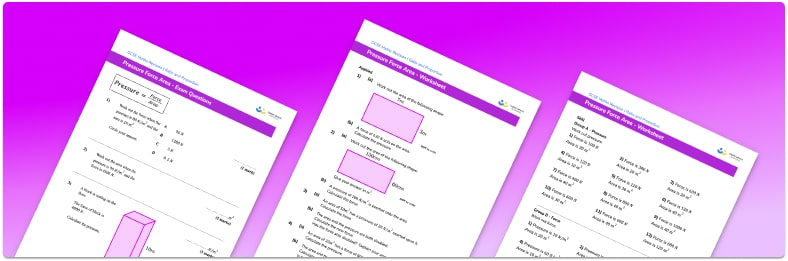• Section 1 of the, pressure, force, area worksheet contains 36 skills-based mass density volume practice questions, in 3 groups to support differentiation
• Section 2 contains 4 applied pressure, force, area questions with a mix of worded problems and deeper problem solving questions
• Section 3 contains 3 foundation and higher level GCSE exam style practice questions including word problems
• Answers and a mark scheme for all exam questions are provided
• Questions follow variation theory with plenty of opportunities for students to work independently at their own level
• All questions are created by fully qualified expert secondary maths teachers
• Suitable for GCSE maths revision for AQA, OCR and Edexcel exam boards

• This field is for validation purposes and should be left unchanged.

You can unsubscribe at any time (each email we send will contain an easy way to unsubscribe). To find out more about how we use your data, see our privacy policy.

### Pressure, force, area at a glance

Pressure is a measure of the amount of force per unit area that a solid object exerts on a surface. Pressure is usually measured in the metric units of Newtons per square metre (N/m²). Sometimes, particularly in physics, pressure is measured in pascals where 1 pascal is equal to 1 N/m²

Calculating pressure can be done using the formula Pressure = Force / Area. The formula can also be used for calculating force or area, given the pressure. This can be done by entering the values we know and rearranging to calculate the unknown value.

Air pressure is the force acting on a surface by the air above it being pulled by gravity.

Looking forward, students can then progress to additional compound measures worksheets and other ratio and proportion worksheets, for example a ratio worksheet or a simplifying and equivalent ratios worksheetFor more teaching and learning support on Ratio and Proportion our GCSE maths lessons provide step by step support for all GCSE maths concepts.

When you have students who require more intensive support our one to one GCSE maths revision programme will match them with the most appropriate tutor. This way we can provide individual students with personalised programmes of study while you continue to teach the rest of your class as a whole group.

Our maths interventions are currently only available for GCSE students, and are not suitable for A level students.

## Related worksheets

Density, Mass, Volume Worksheet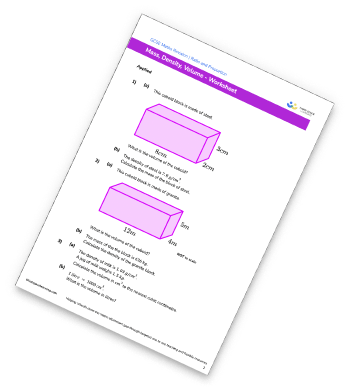Ratio To Fraction Worksheet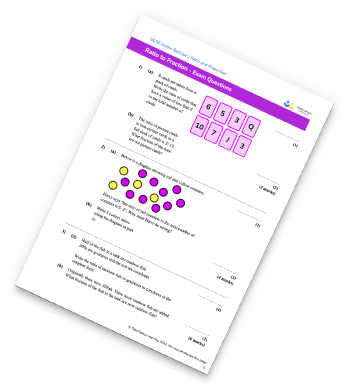Simplifying Ratios Worksheet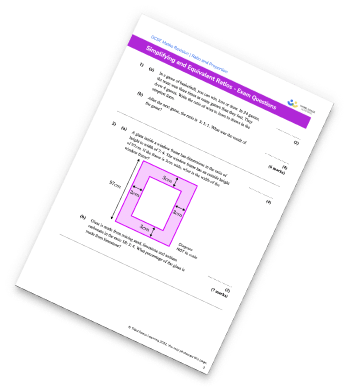Compound Measures Worksheet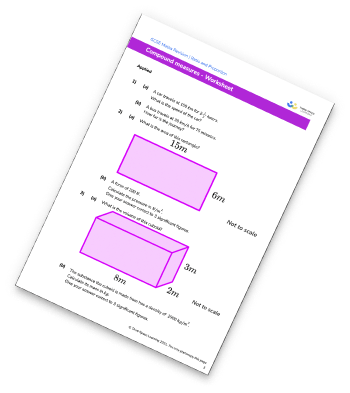## Do you have KS4 students who need more focused attention to succeed at GCSE?There will be students in your class who require individual attention to help them succeed in their maths GCSEs. In a class of 30, it’s not always easy to provide.

Help your students feel confident with exam-style questions and the strategies they’ll need to answer them correctly with our dedicated GCSE maths revision programme.

Lessons are selected to provide support where each student needs it most, and specially-trained GCSE maths tutors adapt the pitch and pace of each lesson. This ensures a personalised revision programme that raises grades and boosts confidence.

Find out more